trustle > EngliSea > Corona Safe Schooling > mathematics > Quick Math Index > 50 Trigonometry

A branch of mathematics that studies relationships between side lengths and angles of trianglesgraph
9097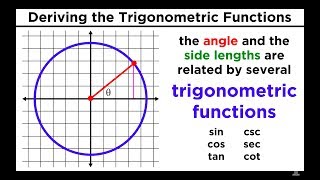02 Trigonometric Functions
Sine, Cosine, Tangent, Cosecant, Secant, and Cotangent
5021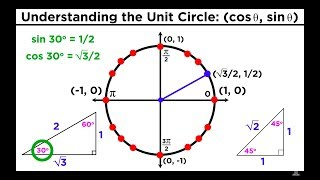03 The Easiest Way to Memorize the Trigonometric Unit Circle
The Easiest Way to Memorize the Trigonometric Unit Circle
5022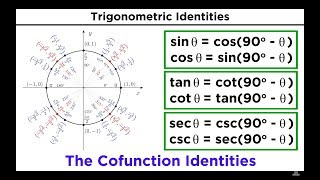04 Basic Trigonometric Identities
Basic Trigonometric Identities: Pythagorean Identities and Cofunction Identities
5107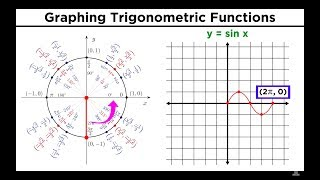05 Graphing Trigonometric Functions
Graphing Trigonometric Functions
5108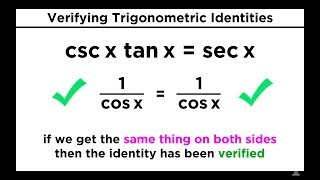07 Verifying Trigonometric Identities
Verifying Trigonometric Identities
511008 Formulas for Trigonometric Functions
Formulas for Trigonometric Functions: Sum/Difference, Double/Half-Angle, Prod-to-Sum/Sum-to-Prod
5111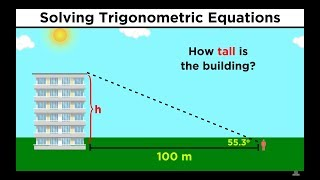09 Solving Trigonometric Equations
Solving Trigonometric Equations
5112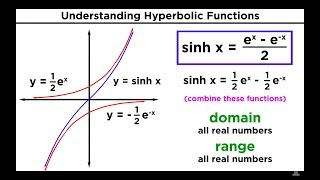Hyperbolic Functions: Definitions, Identities, Derivatives, and Inverses
Hyperbolic Functions: Definitions, Identities, Derivatives, and Inverses
3672Identity
9667Intro to Radians - Nerdstudy-